# Y4 Maths Task- Friday 27th March

Fractions and decimals

You might need to work out on paper and then write it in your reply. If you have a printer, you could print it out and write your answers on there first.

This activity should take around an hour.

Click on the blue link to see the work more clearly.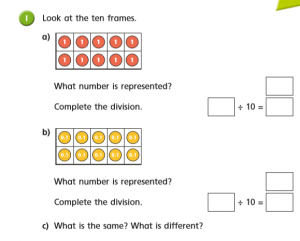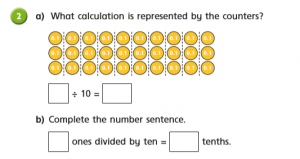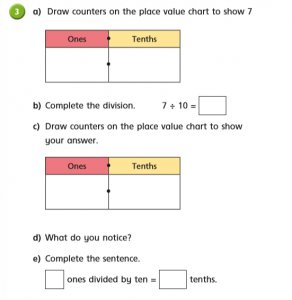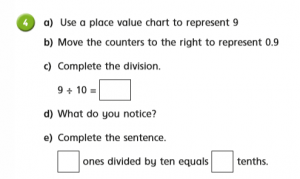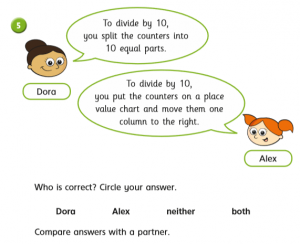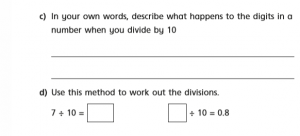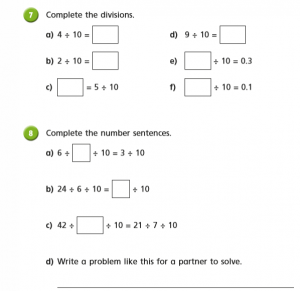Maths lesson 5

### 9 thoughts on “Y4 Maths Task- Friday 27th March”

•27th March 2020 at 10:15 am

1
a] 10/2=5, 10/5=2
b] 1/10=0.1
c] The difference i notice is that one has two calculations for it but the other one has one. I’ve also noticed that on one it has ones but on the other one it has tenths.

2
a] 3/10=0.3
b] Three ones divided by ten equals three tenths.

3
a] 7 ones, 0 tenths
b] 7/10=0.3
c] 0 ones, 3 tenths

4
a] 9 ones, 0 tenths
b] 0 ones, 9 tenths
c] 9/10=0.9
d] I notice that all we had to get nine tenths from nine is just drag the counters over.
e] Nine ones divided by ten equals nine tenths.

5
a] Both are fully correct in this situation.
b] All that happens to them is that they move to the right once and a zero comes in front.
c] 7/10=0.7, 8/10=0.8

6
a] 6/10=0.6
b] zero ones and 6 tenths

7
a] 4/10=0.4
b] 2/10=0.2
c] 0.5=5/10
d] 9/10=0.9
e] 3/10=0.3
f] 1/10=0.1

8
a] 6/1/10=3/10
b] 24/6/10=4/10
c] 42/14/10=21/7/10
d] 63/___/10=49/7/10

•27th March 2020 at 10:51 am

Thank you Franek. Great understanding. Hope you are ok. Mrs Denny

•27th March 2020 at 10:58 am

1.
a) 10
10/10=1

b) 1
1/10=0.1

2.
3/10=0.3

b)
3 ones divided by ten is 3 tenths

3.
a) 7 ones or 7 tenths and 0 tenths or 0 ones

b)
7/10=0.7

c)
seven tenths so 0.1 0.1 0.1 0.1 0.1 0.1 0.1

d)
7 ones divided by ten= 0.7 tenths

4.
a)
9/10=0.9

b)
9 ones divided by ten equals 9 tenths

5.
a) Alex is correct

6.
6 ones
6/10=0.6
0.1 0.1 0.1 0.1 0.1 0.1

c)
if you divide a number 10 you will get a decimal sometimes

d)
7/10=0.7 8/10=0.8

7.
a) 4/10=0.4
b) 2/10=5
c) 0.5= 5/10
d) 9/10=0.9
e) 3/10=0.3
f) 1/10=0.1

8.
a) 6/3/10=3/10
b) 24/6/10=4/10
c)42/7/10=21/7/10

d)
48/_/10=4/10

•27th March 2020 at 11:10 am

It is clear that you have a good understanding of fractions, well done Kristers

•28th March 2020 at 7:34 pm

1.
a) 10/10=1
b) 1/10=0.1

2.
a) 3/10=0.3
b) 3 ones divided by ten = 0.3 tenths

3.
a) 7 tenths
b) 7/10=0.7
c) seven tenths
e) 7 ones divided by ten= 7 tenths

4.
c) 9/10=0.9
e) 9 ones divided by ten equals 9 tenths

5. Alex is correct

6.
a) 6/10=0.6
b) 6 tenths
c) so when you divide ones by 10 you get a decimal
d) 7/10=0.7, 8/10=0.8

7.
a) 4/10=0.4
b) 2/10=0.2
c) 0.5= 5/10
d) 9/10=0.9
e) 3/10=0.3
f) 1/10=0.1

8.
a) 6/2/10=3/10
b) 24/6/10=4/10
c) 42/2/10=21/10
d) 54/9/10=6/10=0.6

•29th March 2020 at 3:12 pm

Brilliant work, well done.

•6th April 2020 at 11:48 am

1a)10:10= 1
b) 1:10=0.1
2) 3: 10=0.3
3 ones divided by ten = 3 tenths
3a) 7 ones
b) 7 : 10 = 0.7
c) 7 tenths
d) On the value chart the counters were moved from ones to tenths.
e)7 ones divided by ten = 7 tenths
4a) 9 ones
b) 9 tenths
c) 9:10=0.9
d) The digit was moved one place to the left.
e) 9 ones divided by ten equals 9 tenths
5) Alex is correct.
c) The digit moved one place to the left.
6a) 6:10= 0.6
b) 6 tenths
7a ) 4:10= 0.4
b) 2:10=0.2
c) 0.5=5:10
d) 9:10= 0.9
e) 3:10=0.3
f) 1:10= 0.1
8 a) 6:2:10=3:10
b) 24:6:10=4:10
c) 42:14:10=21:7:10

•6th April 2020 at 1:49 pm

Great number sense thank you Damian

•